Exercises

# Decimals

In this unit, students will build on their understanding of decimals by working with numbers that include decimals in tenths, hundredths and thousandths place. Adding, subtracting and rounding with decimals will enable them to apply the strategies in new ways and enhance their understanding of the base-ten number system.
This lesson includes 5 printable learning activities.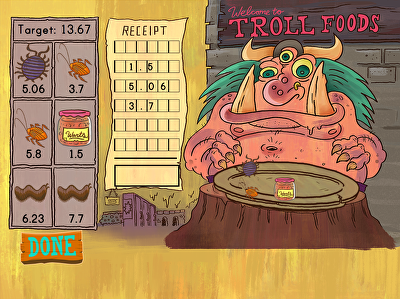Troll is starving, and needs help making a plate at his favorite salad bar. Kids must add food to Troll's plate and correctly align decimal numbers. Once the numbers are aligned, they must add up the total on the receipt, being sure to stay under the maximum target weight on screen. This silly game is perfect practice for students who need help adding numbers to the tenth and hundredth place.

## Addition with Decimal Numbers to the Tenths Place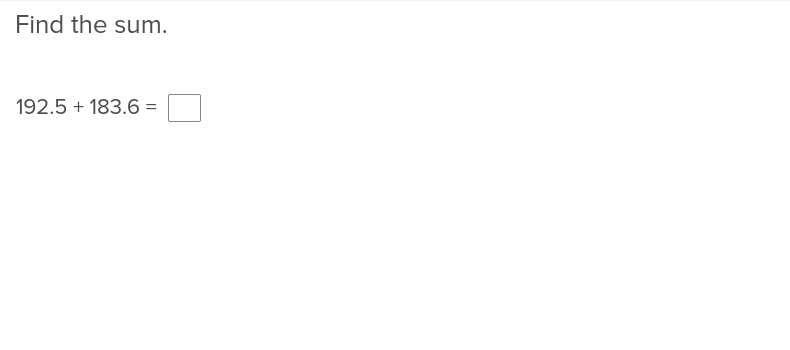Show students how to add decimal numbers to the tenths place with this interactive exercise.

## Addition with Decimal Numbers to the Hundredths Place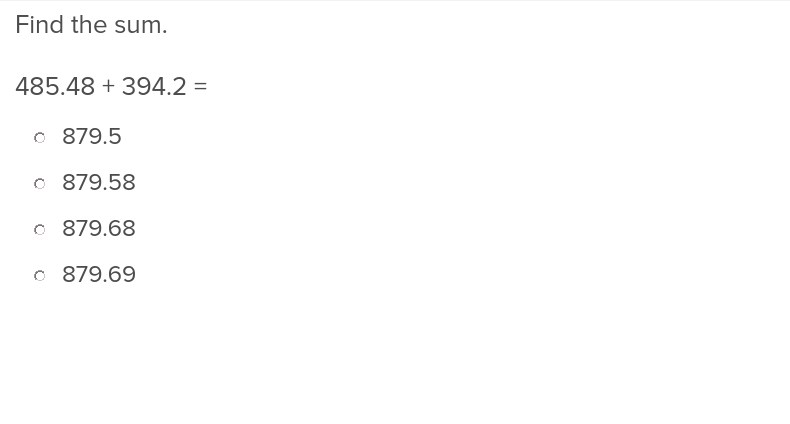Enhancing students’ competency with decimal numbers to the hundredths place is a simple matter of assigning them this exercise.

## Addition with Decimal Numbers to the Thousandths Place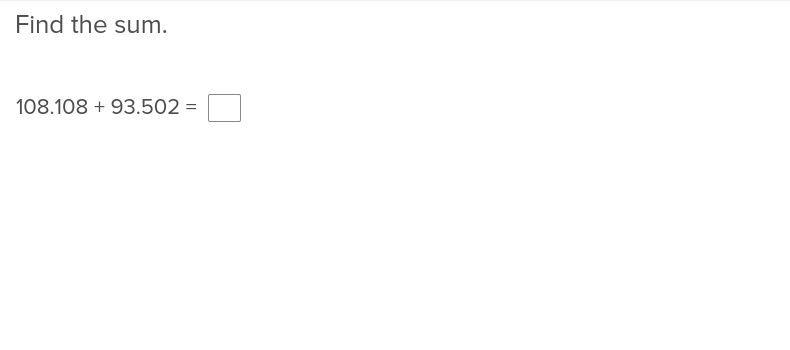Showing students how to add decimal numbers to the thousandths is a snap when you take advantage of this easy to use exercise.

## Subtraction with Decimal Numbers to the Tenths Place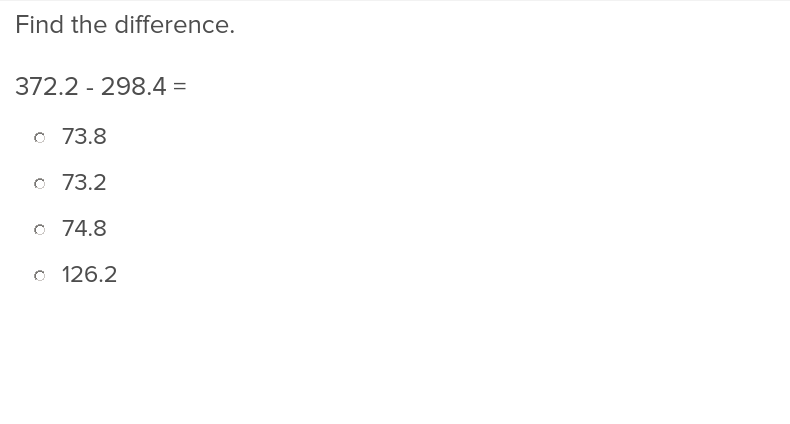This exercise will teach students just how to keep their numbers straight when practicing subtraction with decimals to the tenths place.

## Subtraction with Decimal Numbers to the Hundredths Place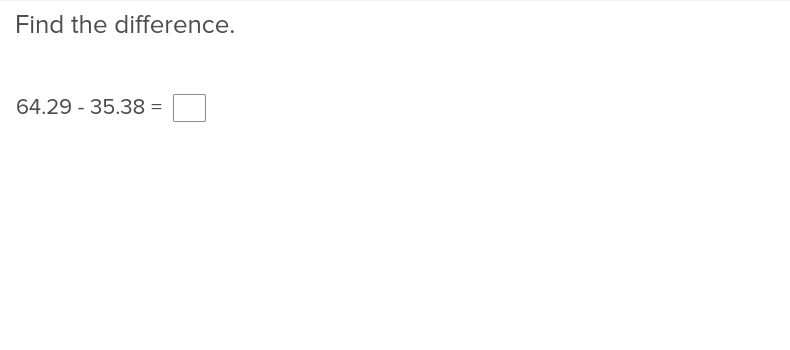Students will be able to work with two digits to the right of the decimal after completing this exercise that shows students how to subtract down to the hundredths place.

## Subtraction with Decimal Numbers to the Thousandths Place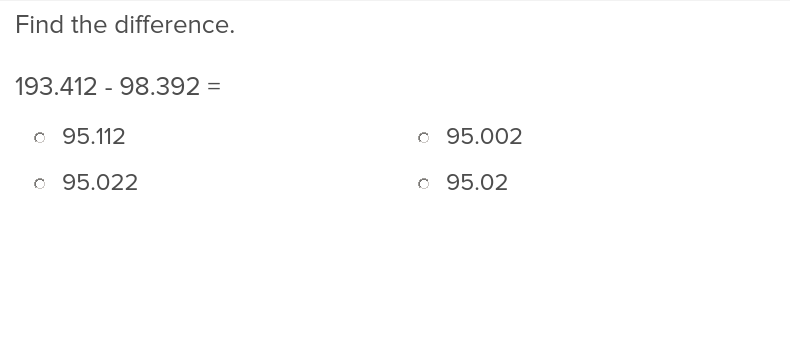This exercise on subtraction to the thousandths place will fine tune students’ attention to detail.

## Rounding Decimal Numbers to the Nearest Tenth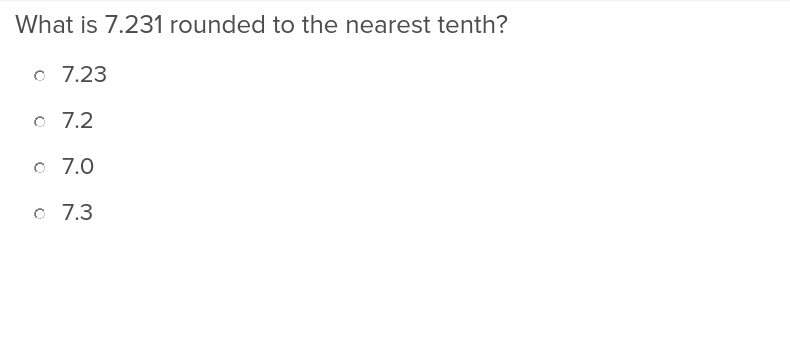Teach students how to simplify math problems by rounding decimal numbers to the tenth with this exercise.

## Rounding Decimal Numbers to the Nearest Hundredth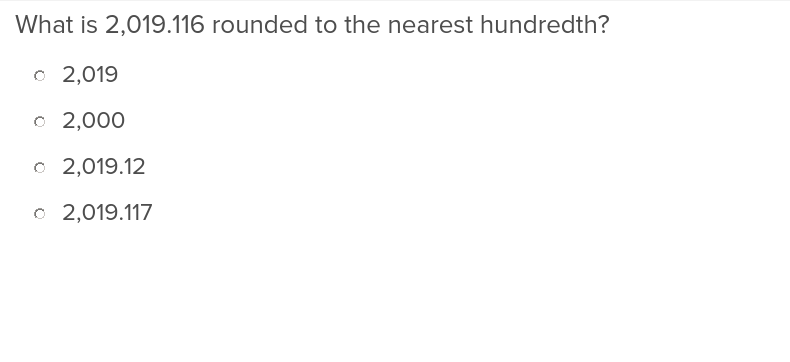Help students take their attention to detail in math problems one step further with this exercise that teaches how to round decimal numbers to the nearest hundredth.

## Rounding Decimal Numbers to the Nearest Thousandth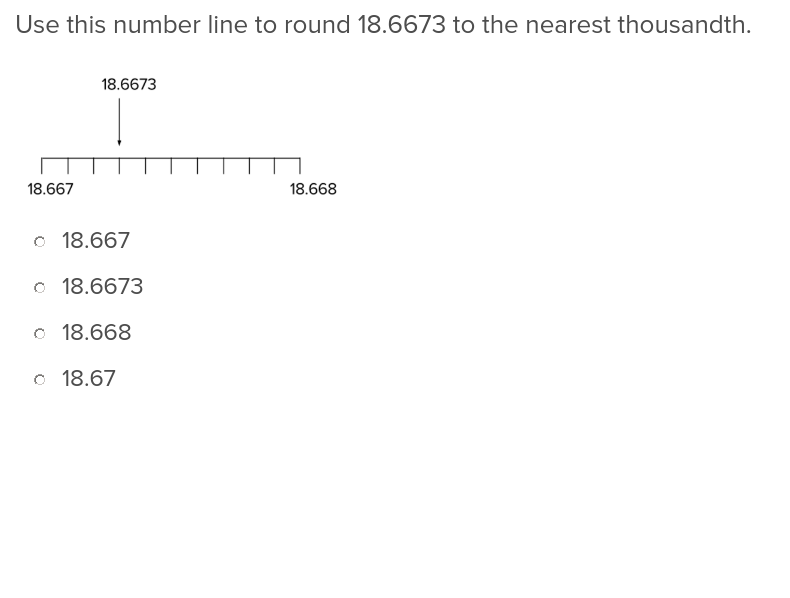When students start to get a little lost in the details, help them simplify problems involving decimals with the ability to round to the nearest thousandth.

Create new collection

0

### New Collection>

0 items

What could we do to improve Education.com?